# Reduced norm

(diff) ← Older revision | Latest revision (diff) | Newer revision → (diff)

Letbe a finite-dimensional central simple algebra over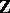. A finite extension fieldofis a splitting field forif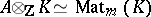as-algebras for some. Hereis the-algebra of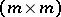-matrices. Choose an isomorphism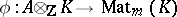. The reduced norm mappingis now defined by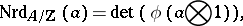and the reduced trace mapping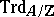is similarly defined byOne checks that the right-hand sides of these equations are indeed in(and not just in) and that the definitions are independent of the choices ofand.

The reduced norm is multiplicative, andis invertible if and only if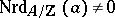. The reduced trace is a homomorphism ofvector spaces, and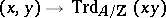defines a non-degenerate bilinear form on.

How to Cite This Entry:
Reduced norm. Encyclopedia of Mathematics. URL: http://encyclopediaofmath.org/index.php?title=Reduced_norm&oldid=13357